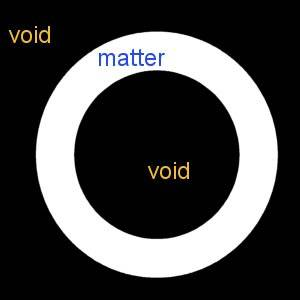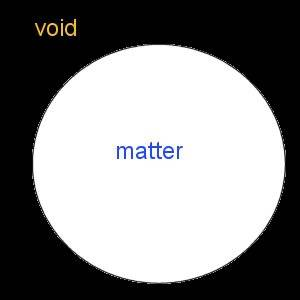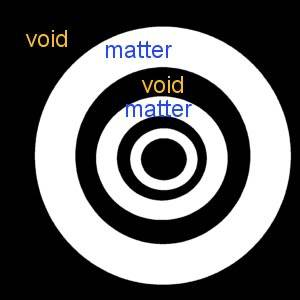# Which universe model is correct

degree451
I’m curious as to what the universe looks like according science theorists. So let’s say the “Big Bang” theory is correct. I have several models of what the universe could look like but I’d like to know which model is the most widely accepted one.

Model A:
Big Bang starts, universe expands, at some point the matter dump from the central point ends. In this model if we were far enough away the universe would resemble a lumpy sphere. All matter in the universe is contained within the relative outer shell of the sphere and the interior of the this sphere is void.Model B:
Big Bang starts, universe expands, the central point continues to dump matter into the universe; it never stops. In this model again the universe is a lumpy sphere and the sphere is solid with matter filling all space from the outer shell all the way back to the center.Model C:
Big Bang starts, universe expands, the central point stops dumping matter into the universe but after a period the central point begins dumping matter into the universe again and this cycle repeats N times. In this model the universe again is a lumpy sphere and each matter “spurt” creates spheres within spheres and the matter from each spurt is separated from the previous cycle’s by a spherical void. Of course if the expansion of one spurt is faster than the previous or slower than its successor then two spurts will eventually catch up with one another eventually banding together.## Answers and Replies

Hello degree451 and welcome to PF!

It's good that you're thinking about the overall structure of the universe -- what's unfortunate is that you seem to be applying your human intuition a little bit too much. You see, you are assuming that there is a static backdrop of space and time and the BB is simply an explosion of matter at the center of this. This is not the case. Space and time were created by this event, and subsequently expanded (not into anything! They intrinsically expanded -- irrespective of anything around them. It's tough to grasp since we always see things expand into empty space, but in differential geometry a surface need not expand into anything for all points on it to get farther away from all the others). So to make these toy models such as yours does not really make any sense within the context of general relativity.

There are many places you can find the current state of what we think the universe as a whole looks like. In brief, we think it is approximately flat, as a sheet of paper is. It could have been positively curved, like the surface of a sphere, or negatively curved, like a saddle, but we observe it to be almost perfectly flat. Furthermore, as far as we can tell, there is no "edge" to the universe, and it is expanding at an accelerating rate.

For links, if you're mathematically inclined:
http://en.wikipedia.org/wiki/Friedmann–Lemaître–Robertson–Walker_metric
Or more generally,
http://en.wikipedia.org/wiki/Shape_of_the_universe

To understand the fundamental concepts of general relativity, like no fixed background of space and time, requites a lot of doing. We have an excellent thread here on the balloon analogy which can help. Also, I'm sure marcus will come along and explain much more elegantly than I have here, but I hope I've at least clarified a bit!

Gold Member
Dearly Missed
... I'm sure marcus will come along and explain ..., but I hope I've at least clarified a bit!

I do appreciate your kind reference, Nabeshin. However you already clarified things plenty elegantly! What's more, we are fortunate just now that quite a few reliable/knowledgeable people are currently active in Cosmology forum. Besides you, there are BAPowell, BCrowell, Chalnoth to name a few, and others. George Jones drops in now and then. Apologies to those I failed to mention.

I can't think anything to add to what you already said. Maybe others can, or perhaps someone will ask followup questions.

LithiumHelios
The general answer to your question will probably come down to the model you choose to solve a number of well known problems with the vanilla cosmological model (I'll throw down some math at the very bottom):

1) Homogeneity problem - why is the Universe so homogeneous on large scales?
2) Flatness problem - Why is the Universe so close to flat? $$\Omega \sim 1$$ is an unstable point in FRW models and we expect divergences from unity as the Universe evolves so in the past the density parameter of the Universe must have been extremely close to unity, is there a mechanism that can do this for us?
3) Relic particle problem - GUT theories generically predict fun particles such as magnetic monopoles and other neat things but observationally we don't get these, is there a mechanism to dilute away these numbers?
4) Horizon/Causality problem - Why do e.g. patches of the CMB on opposite sides of the sky have the same temperature? Implies a thermal equilibrium but in vanilla FRW models if we rewind the history of the Universe we observe that only patches of the sky ~ separated by a degree may have been in causal contact - is there a way around this?

In order to solve the above (and to eventually answer your question) it was suggested by Guth, Linde, Starobinksy etc that we could invoke an inflationary epoch in the early Universe as an addition to the LCDM cosmological models.

The canonical example for an inflationary model is a single free scalar field with canonical kinetic terms that is minimally coupled to gravity initially existing in some excited energy state such that it's potential energy dominates it's kinetic energy. A scalar field is just a function with a numerical value at each point in space and time. A good example of something you'd know is the temperature in a room. It has a value at each point in space and time (e.g. 10 degrees by the door but 5 degrees by the window etc). Canonical kinetic terms is more technically involved but ~ relates to how the field propagates and shouldn't worry you too much. Minimally coupled is another technical term and it just means that we assume Einstein General Relativity holds and that nothing strange is happening with gravity and our field. An excited energy state just means that we do not want our field at the minimum possible potential that it may have - e.g. in our temperature analogy if the temperature were absolute zero everywhere then this would be a trivial configuration and would be somewhat meaningless. Potential energy of the field can be related to classical analogies such as the potential energy in a gravitational potential and kinetic energy ~ relates to how the field evolves along it's potential, V, in time.

Under these assumptions we find that the scalar field, initially displaced from it's true vacuum state, is allowed to slowly roll down some flat potential (required for a sustained period inflation as if the kinetic energy ~ potential energy then inflation will terminate) towards it's true minima. The fact that the potential energy dominates allows the scalar field to act as a source of negative pressure driving the expansion of the Universe.

So the key ingredient is that the scalar field above is quantum mechanical in nature and this means that we can have quantum fluctuations about some homogeneous background. These quantum mechanical fluctuations will source primordial density perturbations that can then undergo gravitational collapse to form large scale structure, CMB etc. So the basic picture in this model is that quantum mechanical fluctuations seed density perturbations that are then stretched out by the expansion of the Universe, driven by our hypothetical quantum scalar field (the inflaton), to astrophysical scales and this process occurs throughout the inflationary epoch.

So inflation solves the horizon problem by initially generating things in causal contact before dragging them out the horizon, it wipes out any relic curvature etc and drives the Universe to an ~ flat configuration, it dilutes relic particles due to the rapid expansion and the quantum fluctuations provide a mechanism to generate structure in the Universe and as the perturbations occur throughout the whole process we get a Universe that is ~ homogeneous on large scales. Local inhomogeneity (i.e. the solar system is distinct to the galaxy as a whole) arises due to gravitational collapse - slightly overdense regions could be allowed to undergo collapse if they satisfy the criteria for gravitational instability etc.

So long story short the canonical model for inflation and structure formation is not quite any of the models you've posted above. It's also important to realise that the Universe doesn't have a center and that this inflationary expansion occurs at all points. The scalar field is just a quantum field with a value for the potential energy at each point in spacetime etc.

Math-y Stuff (other folk may find this helpful too but it's well covered in many textbooks but hey...):
Minimally coupled single free scalar field with canonical kinetic terms:

$$S = \int d^4 x \sqrt{-g} \left[ \frac{M_{\rm{pl}}}{2}R - \frac{1}{2}g^{\mu \nu} \nabla_{\mu} \phi \nabla_{\nu} \phi - V(\phi) \right]$$

Stress-Energy Tensor:

$$T_{\mu \nu} = \frac{-2}{\sqrt{-g}} \frac{\partial}{\partial g^{\mu \nu}} ( \sqrt{-g} \mathcal{L}_{\phi})$$

Which will give us:

$$T_{\mu \nu} = \partial_{\mu} \phi \partial_{\nu} \phi - \frac{1}{2} g_{\mu \nu} \partial_{\omega} \phi \partial^{\omega} \phi - g_{\mu \nu} V(\phi)$$

where for simplicity I have let $$\nabla_{\nu} \rightarrow \partial_{\nu}$$.

So now we can solve for the energy density and pressure of the field by assuming that we have an ideal fluid giving us a stress-energy tensor of:

$$T_{\mu \nu} = (p + \rho)U_{\mu} U_{\nu} - p g_{\mu \nu}$$ if $$g_{\mu \nu} = (-,+,+,+)$$.

and $$T_{0 0} = \rho$$, $$T_{jj} = p_j$$ if we assume an observer at rest: $$U_{\mu} = (1,0,0,0)$$. Hence the energy density and pressure will be:

$$\rho_{\phi} = \frac{1}{2} \dot{\phi}^2 + V(\phi)$$
$$p_{\phi} = \frac{1}{2} \dot{\phi}^2 - V(\phi)$$.

Now we assumed that the potential energy dominated the kinetic energy so: $$V \gt \frac{1}{2} \dot{\phi}^2$$. Hence:

$$p \sim - V(\phi) \sim - \rho$$.

We can now use this result along with the time-time solution to Einsteins equation (which is just Friedmann's equation) to give:

$$H^2 = \frac{8 \pi G}{3} \rho = \frac{8 \pi G}{3} V(\phi)$$. We can also find thath the scale factor describing ~ how the Universe changes in size with time will be given by: $$a(t) \sim e^{H t}$$. Hence we end up with exponential expansion!

So that's the general gist of inflation. Hope that answers the question. I would post a little something about the quantum fluctuations but it's getting a bit long for a lunch break, sorry. All we really mean is that: $$\phi (t,x) = \bar{\phi}(t) + \delta \phi (t,x)$$ where $$\delta \phi$$ is some quantum fluctuation about the mean background.

Really should get back to working now but if you have any follow ups then I'm sure someone can answer them. Sorry if that's too technical or not technical enough, always hard to judge what background people have but hopefully theres enough of both to answer the question :)

NB/ I really hope I didn't say something stupid above or mess up minus signs or whatever too much, sorry if there's some loose statements :S

Last edited: